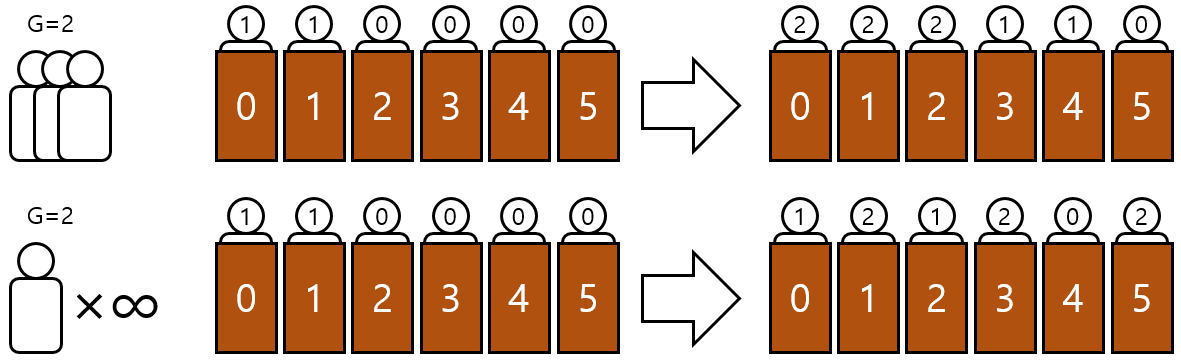시간 제한메모리 제한제출정답맞힌 사람정답 비율
1.5 초 (추가 시간 없음) 1024 MB300534616.547%

## 문제

Hilbert's Hotel has infinitely many rooms, numbered 0, 1, 2, ⋯. At most one guest occupies each room. Since people tend to check-in in groups, the hotel has a group counter variable $G$.

Hilbert's Hotel had a grand opening today. Soon after, infinitely many people arrived at once, filling every room in the hotel. All guests got the group number 0, and $G$ is set to 1.

Ironically, the hotel can accept more guests even though every room is filled:

• If $k$ ($k \geq 1$) people arrive at the hotel, then for each room number $x$, the guest in room $x$ moves to room $x+k$. After that, the new guests fill all the rooms from 0 to $k-1$.
• If infinitely many people arrive at the hotel, then for each room number $x$, the guest in room $x$ moves to room $2x$. After that, the new guests fill all the rooms with odd numbers.You have to write a program to process the following queries:

• 1 k - If $k \geq 1$, then $k$ people arrive at the hotel. If $k = 0$, then infinitely many people arrive at the hotel. Assign the group number $G$ to the new guests, and then increment $G$ by 1.
• 2 g x - Find the $x$-th smallest room number that contains a guest with the group number $g$. Output it modulo $10^9 + 7$, followed by a newline.
• 3 x - Output the group number of the guest in room $x$, followed by a newline.

## 입력

In the first line, an integer $Q$ ($1 \leq Q \leq 300\,000$) denoting the number of queries is given. Each of the next lines contains a query. All numbers in the queries are integers.

• For the 1 k queries, $0 \leq k \leq 10^9$.
• For the 2 g x queries, $g < G$, $1 \leq x \leq 10^9$, and at least $x$ guests have the group number $g$.
• For the 3 x queries, $0 \leq x \leq 10^9$.

## 출력

Process all queries and output as required. It is guaranteed that the output is not empty.

## 예제 입력 1

10
3 0
1 3
2 1 2
1 0
3 10
2 2 5
1 5
1 0
3 5
2 3 3


## 예제 출력 1

0
1
0
9
4
4


## 노트

If you know about "cardinals," please assume that "infinite" refers only to "countably infinite." If you don't know about it, then you don't have to worry.